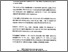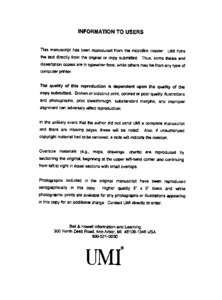Title:

# A finite element method for the fully-coupled magnetohydrodynamics

Ben Salah, Nizar (1999) A finite element method for the fully-coupled magnetohydrodynamics. PhD thesis, Concordia University.Preview
Text (application/pdf)
NQ43584.pdf
4MB

## Abstract

This thesis presents a finite element method for the solution of three-dimensional magnetohydrodynamic flows. The physics of such flows is governed by the Navier-Stokes equations, which are extended to account for the Lorentz forces, and by Maxwell equations from which the magnetic induction equation is obtained. Magnetohydrodynamic problems are, thus, interdisciplinary and coupled in a two-way fashion, since the effect of the velocity field appears in the magnetic transport equations, and the interaction between the electric current and the magnetic field appears in the momentum transport equations. Such a complex problem has to be carefully addressed and we choose to tackle it in a two-step fashion. In the first step, the magnetic problem is addressed. A conservative stabilized finite element method is proposed, taking into account, and this for the first time, the explicit respect of the free-divergence constraint. The introduction of this equation would normally lead to an over-determined system of equations. The introduction of a new scalar variable circumvents this overdetermination by giving rise to an equivalent system of equations which is not over-determined. This scalar is interpreted as the Lagrange multiplier of the free-divergence constraint on the magnetic field. In the second step, the fully-coupled problem is tackled. The Navier-Stokes equations are extended to account for the electromagnetic Lorentz forces. Since the simultaneous solution of all the fluid and the magnetic variables would require huge computational requirements, a segregated algorithm is proposed for the solution of the coupled equations. Versions of this algorithm, taking into account the relative intensity of the magnetic diffusion to the magnetic convection, have been developed. It is seen from the numerical tests that updating the magnetic field at each time step iteration is enough for good convergence even for high magnetic Reynolds numbers Re m . Numerical results are obtained for both the decoupled magnetic problem and the coupled magnetohydrodynamic problem. The first tests address the stability and the accuracy of the finite element formulations that are developed throughout this thesis, while other tests concern 1D, 2D and 3D typical benchmarks in the MHD context. Results are satisfying showing good agreement with analytical solutions when available, putting into evidence the physical effects of the electromagnetic forces and the physical mechanisms governing the magnetic quantities and underlying the robustness of the developed segregated algorithm.

Divisions: Concordia University > Gina Cody School of Engineering and Computer Science > Mechanical and Industrial Engineering Thesis (PhD) Ben Salah, Nizar xvii, 139 leaves : ill. (some col.) ; 29 cm. Concordia University Ph. D. Mechanical and Industrial Engineering 1999 Habashi, Wagdi G. 903 Concordia University Library 27 Aug 2009 17:15 18 Jan 2018 17:15 http://clues.concordia.ca/search/c?SEARC...
All items in Spectrum are protected by copyright, with all rights reserved. The use of items is governed by Spectrum's terms of access.

Repository Staff Only: item control pageResearch related to the current document (at the CORE website)
Back to top